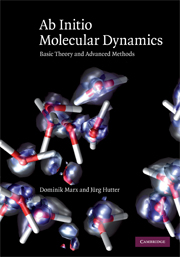Monday, September 10, 2012

Ab Initio Molecular Dynamics Simulation Methods in Chemistry PDF LECTURE SLIDESAb Initio Molecular Dynamics Simulation Methods in Chemistry

Instructor: Stefano Baroni
Textbook: Ab Initio Molecular Dynamics Simulation Methods in Chemistry

Density-functional pertubation theory [pdf]

Molecular structure and dynamics with DFT [pdf]

Computer simulation of thermally activated processes [pdf]

Structural Optimization [pdf]

PWSCF First Examples [pdf]

Rare Events [pdf]

Ab Initio Molecular Dynamics: Part One [pdf]

Ab Initio Molecular Dynamics: Part Two [pdf]

Solution of the Electronic Schroedinger Equation

Using Basis Sets to Solve the Hartree-Fock-Equations [pdf]

Using Basis Sets to Solve the Electronic Schrodinger Equation with Electron Correlation [pdf]

Exercise: Systematic Improvement of Basic Sets [pdf]

Lab: Quantum Chemistry with GAMESS [pdf]

GAMESS and MACMOLPLT [pdf ,ppt]

Lab: macmolplt.tar.gz [.gz]

Introduction into DFTB [pdf]

Introduction into DFTB [pdf]

Introduction into DFTB [pdf]

Approximate methods for large molecular systems [ppt]

DFT and VdV interactions [ppt]

QM/MM Calculations and Applications to Biophysics [ppt

Quantum Monte Carlo Methods [pdf]

Quantum Monte Carlo Methods: recent developments and applications [pdf]

Quantum Monte Carlo lab notes [pdf]

Conical Intersections [pdf, ppt]

Electronic Structure for Excited States [pdf, ppt]

Lab: Multi-reference [pdf, doc]

Introduction to Car-Parrinello Ab Initio MD [pdf]

Equations of Motion and Numerical Integration [pdf]

Equilibrium Statistical Mechanics [pdf]

Lecture Notes: Introduction to AIMD Simulations [pdf]

Ab Initio Multiple Spawning Dynamics [pdf]

Ab Initio Quantum Molecular Dynamics [pdf]

Insights for Light-Driven Molecular Devices from Ab Initio Multiple Spawning Excited-State Dynamics of Organic and Biological Chromophores [pdf]

Introduction to Full Multiple Spawning using MOPAC [pdf

Reactive Empirical Force Fields [pdf, ppt]

Lab: deformation of nanoscale materials [pdf]

Molecular dynamics modeling of thermal and mechanical properties [pdf, ppt]